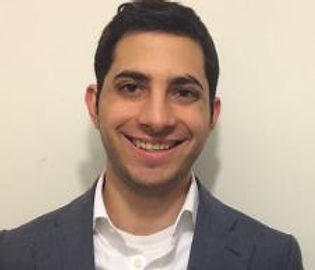top of page## GRE 93%## NYC High School AP Instructor

I am a College graduate (Yeshiva University) who majored in philosophy and math. I have also received a masters in math (Yeshiva University).

For the past five years I have been tutoring college and post high school students in logic, set theory, epistemology, metaphysics, modal logic, philosophy of law, algebra 1 and 2, calc 1, 2 and 3. Advanced calc 1 and 2, modern algebra, ACT, SAT and many other subjects. I'm currently also teaching two geometry class in high school.

When tutoring I prefer doing a very small amount of questions very carefully so that students understand the intuitions behind the math and don’t just memorize steps for how to solve problems. First, I believe that this helps students memorize the steps, makes the learning experience much more entertaining and builds mathematical creativity. In fact, after a deep intuition of the math is achieved one should be able to come up with the steps for solving a problem on one’s own. You might think that this approach takes a lot of time and provides superfluous information, especially for those who do not want to understand math and just want to use it (or pass a test), but it is my honest belief that this approach will not only help students learn math in a deeper manner but is also the fastest and most efficient way for memorizing formulas and steps for solving mathematical problems.

Often students believe that they understand the mathematical concepts very well but struggle with specific questions. This normally happens when details of the concepts are missing and so my job is to try and figure out what parts of the mathematical concepts are being misunderstood and then explain the concepts in a more nuanced manner. My ultimate goal is to teach students what mistakes they made without actually pointing out the mistakes. I do this by strengthening their understanding and asking them questions about their approach for solving the problem, questions which, ideally, motivates their realization of the mistakes.

I love math and I love explaining math to people.

###### Subjects

• Algebra 1, Algebra 2, Calculus, Geometry, Prealgebra, Precalculus, SAT Math, Differential Equations, Discrete Math,  Linear Algebra, Logic,

• ACT Math, GRE, SAT Math

bottom of page